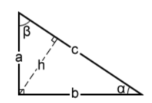# Pythagorean Theorem Calculator (and other geometry + math)

Text Blaze is great for doing math and making calculations for you. It can easily handle all the basic math (addition, multiplication, etc...) that you throw it. Snippets that automatically do math for you like this are the foundation of how many users use Text Blaze.

But it can also handle some pretty complex calculations. Using the formula command with math operators alongside math functions allows you to complete almost any calculation using Text Blaze. Here's two examples:

## Pythagorean Theorem Calculator and Right Triangle Calculator

Most people probably remember being taught the Pythagorean Theorem in high school. It's an extremely useful tool in calculating the dimensions of a right triangle and has uses in construction, design, and fabrication outside of the classroom.

This snippet can calculate the Pythagorean Theorem as well as several other properties of a right triangle instantly, including:

• the length of the hypotenuse, using the length of the two legs.
• the length of a leg, using the length of the hypotenuse and the other leg.
• the interior angles of the triangle.
• the area of the triangle.
• the perimeter of the triangle.
• the height of the triangle.

{note}Enter two values below to solve for the third.

a: {formtext: name=a}

b: {formtext: name=b}

c: {formtext: name=c}{letters=yes if testregex(a, "[a-z]") OR testregex(b, "[a-z]") OR testregex(c, "[a-z]") else no}{impossible=yes if a<>"" and b="" and c<>"" and (a>c or a=c) else yes if a="" and b<>"" and c<>"" and (b>c or b=c) else no}
{endnote}
{if: letters}{error: Only enter numbers}{elseif: impossible}{error: The value for the hypotenuse must be larger than the legs}{elseif: a<>"" and b<>"" and c<>"" and not letters and not impossible}{error: Please enter only two starting values}{endif}{if: a<>"" and b<>"" and c="" and not letters and not impossible}{avalue=a}{bvalue=b}{cvalue=sqrt((a^2)+(b^2))}c = {=cvalue}, or √ ({=(a^2)+(b^2)}){elseif: a<>"" and b="" and c<>"" and not letters and not impossible}{avalue=a}{bvalue=sqrt((c^2)-(a^2))}{cvalue=c}b = {=bvalue}, or √ ({=(c^2)-(a^2)}){elseif: a="" and b<>"" and c<>"" and not letters and not impossible}{avalue=sqrt((c^2)-(b^2))}{bvalue=b}{cvalue=c}a = {=avalue}, or √ ({=(c^2)-(b^2)}){endif}
Area: {=catch((avaluebvalue)/2, "Provide values to calculate")}
Perimeter: {=catch(avalue+bvalue+cvalue, "Provide values to calculate")}
∠α: {=catch(asin(avalue/cvalue), "Provide values to calculate")}° or {=catch((asin(avalue/cvalue))
∠β: {=catch(asin(bvalue/cvalue), "Provide values to calculate")}° or {=catch((asin(bvalue/cvalue))*(3.1415926535/180), "null")} rads
Height: {=catch(((avalue*bvalue)/cvalue), "Provide values to calculate")}

Refer to this image for calculations:## Percentage Change Between Two Values

I've always struggled with thinking about the change between two numbers in terms of a percentage. It's easy to calculate the number difference between two values, but much trickier to express that as a percentage of change.

This snippet lets you input the starting and ending values, and automatically calculates the percentage change between the two. Whether that's an increase or decrease, the snippet will output how much the starting number changed to become the ending number in terms of a percentage.

{note}Enter starting amount: {formtext: name=starting}

Enter ending amount: {formtext: name=ending}
{change=((ending-starting)/starting)}
{endnote}{if: starting="" or ending=""}{error: Add both starting and ending values to calculate.}{else}{if: change>0}Percentage increase: {=change; format=.2%}{elseif: change<0}Percentage decrease: {=change; format=.2%}{elseif: change=0}The amounts are the same.{endif}{endif}

These are just a few examples of powerful math that you can complete with Text Blaze. If you've got other common calculations that you need to do, share below!

If you've already built a calculator I'd love to see it, but you can also request something here that would be particularly helpful to you.